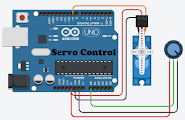How To Control Servo Motor Using ArduinoIn previous tutorials we learnt "How to use a pushbutton with Arduino" and "How to control DC motor with Arduino". In this article we will see how to control Servo Motors. Servo motors are very useful in many projects, mostly robotic. Before we can control one we have to know what a Servo motor is and how it works.

Introduction To Servo Motor:

There are many types of servo motors in the market in a variety of form factors. But all work in a similar fashion. Unlike a DC motor which rotates 360° a Servo motor just travels 180° and has a gear system which increases the torque.

In above image you can see the internals of a standard hobby servo. It consists of 3 important parts, the motor, control circuit and drive gears. All of these are enclosed in a case. The circuit is a closed loop motor controller with a feed back potentiometer. The potentiometer sends feedback to the circuit which tells the current position of the servo shaft.

Pinouts:

As you can see in the image Servo has 3 pins. Signal, Vcc and Gnd, these pins can be recognized based on the colour of the wire. Usually Orange/Yellow is the signal wire, Red is Vcc and Black is Gnd.

Now that you are familiar with pins of servo we can move on to see how we can control a servo using Arduino. Before that here are some things you will need:

Requirement:

Now that you have gathered all the required components, we can move forward to control the servo.

Controlling Servo Using Arduino:

To control servo we need PWM signals, PWM stands for Pulse Width Modulation. In PWM a digital pin is used to generate a square wave. It is a signal created by high and low pulses with an equal interval of time. The duration of on time / High signal is called as Pulse Width and controlling the duration can create different frequencies.

Arduino has 6 PWM pins (3,5,6,9,10 & 11). As arduino is a 8-bit controller its PWM is 0-255, 0 being lowest and 255 being highest. When the output is 255 the pin is at 5v constant, for 127 it is 2.5v and so on.

To control the Servo we will use this feature of the arduino. So lets start with something simple.

1. Simple Servo Control With Arduino:

First connect the servo to arduino as shown in the figure. The Vcc is connected to 5v, Gnd is connected to Gnd and Signal pin is connected to pin 9. The servo is powered directly from arduino but if you are planning to use more than one servo, I suggest using an external power supply. For more information of power requirements of your servo check it's data sheet.

Now refer the code bellow:

#include<Servo.h> //import the servo library

Servo myServo; //set servo variable as myServo

void setup()
{
myServo.attach(9); //connect servo signal to pin 9
}

void loop()

{
for(int i=0; i <= 180; i++) //goes 0 to 180 real quick ;p
{
myServo.write(i); //writes the value to turn servo
delay(50); //50ms delay
}

for(int i=0; i>= 0; i--) //goes form 180 to 0
{
myServo.write(i); //writes the value to turn servo
delay(50); //50ms delay
}
}

Upload this code to uno. You will notice the servo sweeps from end to end.

This code is best for checking if a servo is working. But for most projects we need a precise way to control servo angle. For that we will use a potentiometer.

2. Controlling Servo Using Potentiometer:

In this circuit the servo connections are similar to previous one. Here we have added a 10k ohm potentiometer (variable resistor). The central terminal of pot is connected to A0 pin, other two pins are connected to 5v and Gnd. The pot will be used to generate analog values which we will map to the servo. Thus the change in resistance of the potentiometer will help us control the movement of servo precisely.

Lets take a look at the code:

#include<Servo.h>

Servo myServo;
int potPin = 0; //Set a variable named potPin as A0

void setup()
{
myServo.attach(9);
}

void loop()
{
int val = analogRead(potPin); // read the pot value,save it in val
val = map(val, 0, 1023, 0, 180); //scale the pot readings to 0-180
myServo.write(val); //set the servo position according to val
delay(50); //50ms delay
}

Upload the code to arduino and you should see the servo move to a specific position. It depends on the position of potentiometer. Now turning the pot will move the servo head.

The above method combined with other projects can be used for many applications like RC toys, robots and much more. We will see some projects related to it in future.

That's all for this tutorial. If you have any doubts or questions, feel free to ask in the comment. If you like my tutorials do follow for more.

See you in next tutorial, until then keep experimenting... ;)